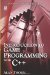1.2 Arithmetic

1.2 Arithmetic

Nowadays, people often use the word "arithmetic" to mean mathematics in general. However, arithmetic actually refers to the basic operations that occur to numbers, such as addition, subtraction, multiplication, and division. Using these operations together can form expressions in which various values can be represented. These operations will now be considered.

If I am the owner of one tomato, and after searching through the garden I find two more tomatoes, I will have increased my total of tomatoes to three. This process of combining two or more quantities is called addition, and the result of an addition is called the sum. So, the sum of 1 + 2 is 3.

1.2.2 Subtraction

If after collecting three tomatoes, someone comes along and steals one of my tomatoes, I shall be left with two. The process of reducing one quantity by another is called subtraction, and the result of subtraction is called the difference. So, the difference of 31 is 2. Subtraction is the opposite (or inverse) of addition.

1.2.3 Multiplication

Increasing a quantity in terms of multiples means to multiply a quantity. For example, 2 × 3 is the same as 3 + 3 + 3. And 4 × 5 is the same as 5 + 5 + 5 + 5. The result of multiplication is called the product. So, the product of 7 × 3 is 21.

1.2.4 Division

Division is the opposite (or inverse) of multiplication. Rather than increasing a quantity, division is the process of determining how many times one quantity fits into another. The result of division is called the quotient. The quantity to be divided is called the dividend, and the quantity it's divided by is called the divisor. So the quotient of 8 ÷ 2 is 4, because 2 (the divisor) fits into 8 (the dividend) exactly four times.Introduction to Game Programming with C++ (Wordware Game Developers Library)
ISBN: 1598220322
EAN: 2147483647
Year: 2007
Pages: 225
Authors: Alan Thorn

Similar book on Amazon